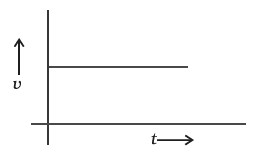#### From the given v – t graph (Figure), it can be inferred that the object is(a) in uniform motion(b) at rest(c) in non-uniform motion(d) moving with uniform accelerationAns. A

Sol.

If we observe the graph carefully we can see that at every time, the value of velocity remains constant.

Hence the acceleration or retardation is zero as a speed is not changing.

The velocity is constant but it is non-0 hence particle must be moving with uniform velocity.

The motion of such type is called uniform motion.

hence the correct option is option A